Time: 3 Hours                                                                                                     Max. Marks: 100

NOTE: There are 9 Questions in all.

·      Question 1 is compulsory and carries 20 marks. Answer to Q. 1. must be written in the space provided for it in the answer book supplied and nowhere else.

·      Out of the remaining EIGHT Questions answer any FIVE Questions. Each question carries 16 marks.

·      Any required data not explicitly given, may be suitably assumed and stated.

Q.1       Choose the correct or best alternative in the following:                                         (2x10)

a.       The smallest increment of the input quantity to which the measuring system responds is called_______.

(A)  sensitivity                                      (B)  precision

(C)    repeatable                                   (D)  resolution

b.      Considering in RTD, if the resistances at temperatures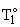c and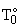c are respectively 136.8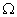and 120.0, also the temperature coefficient of resistance is 0.004, then the difference in temperature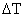is ______.

(A)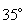(B)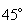(C)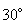(D)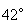c.   The bridge that is useful for measurement of unknown inductance is called ____bridge.

(A) Schering                                        (B) Maxwell

(C) Hay’s                                            (D)  Wien

d.   In a CRO the delay line is situated between________.

(A)    time base generator and the horizontal amplifier.

(B)    vertical amplifier and the vertical deflecting plates.

(C)  trigger circuit and the time base generator.

(D)  horizontal amplifier and the horizontal deflecting plates.

e.   The magnetic flux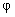is analogous to ______in the electric circuit and is related to the m.m. f. (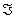) and the reluctance R as______.

(A)     Voltage,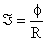(B)     Current,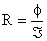(C)     Current,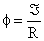(D)    Voltage,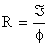f.    Considering a strain gauge, the unit change in length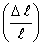is denoted as strain,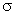and obeys the relation= ______, where s is the stress in Kg/om2 and E is young’s modulus, also in Kg/cm2

(A)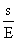(B)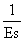(C)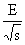(D)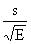g.   When the frequency of a sinusoidal signal applied to the horizontal deflecting plates is twice that of the frequency of a simutar signal applied to the vertical deflecting plates, the Lissajous pattern obtained on the CRT will be_____.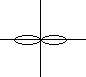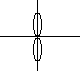(A)                                                         (B)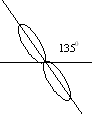(C)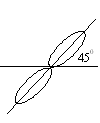(D)

h.   In respect of receiver measurements, the ability to pick up weak signals is called_______.

(A)    Noise figure                                  (B) selectivity

(C) Image response                             (D) sensitivity

i.    The A/D converter which resolves a five-digit number in (2)5 comparisons is called a ______A/D converter.

(A)   Counter type                                (B) successive approximation

(C)  continuous                                    (D) simultaneous

j.    The Hall effect transducer is useful for measuring ____in conductors without interruptor of the circuit.

(A)    Only D.C. current

(B)    Only A.C. current

(C)    Neither DC nor A.C. currents

(D) both D.C. and A.C. currents

Answer any FIVE Questions out of EIGHT Questions.

Each question carries 16 marks.

Q.2     a.   Explain the principle and function of a pressure Inductive transducer, with the help of a schematic diagram.                                                             (6)

b.   Give the circuit-cum-schematic diagram of a simple servo-system and describe its operation.                                                                     (10)

Q.3     a.   Explain the principle of a Digital Frequency meter                                               (8)

b.   Describe a method for the measurement of flux by means of induced emf.            (8)

Q.4     a.   Describe the following parts of a CRO (i) Direct probes (1:1) (ii) Passive voltage (High z) Probes (iii) Active probes.                                                (9)

b.   Give the circuit diagram of a Hay’s bridge and describe its uses. Also write its balance equation.                                                                (7)

Q.5     a.   Distinguish between a sensor and a transducer. Also name three each, of passive and active transducers.                                                                (7)

b.   Give detailed explanation for the terms: (i) Gross errors. (ii) Systematic errors and (iii) Random errors.                                                                   (9)

Q.6     a.   Describe a thermocouple based instrument for measuring temperature.                (8)

b.   A resistance strain gauge with a gauge factor of 2.5 is cemented to a steel member, which is subjected to a strain of 1.6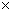10-6. If the original resistance value is 140, calculate the change in resistance of the gauge.                                                           (8)

Q.7     a.   Explain the principle and describe the method of F.M. receiver alignment.         (10)

b.   Briefly explain: sensitivity, image rejection and noise figure as applied to radio receivers.                 (6)

Q.8           Write notes an any TWO of the following:-

(I)                 One type of harmonic distortion analyser based on fundamental suppression.

(II)              RF wattmeter based on a toriod core transformer.

(III)            Sample-and-hold circuit.                                                                      (16)

Q.9         a.     Describe a counter-type A/D converter with multiplexed input.                      (8)

b.    Describe the thermal method for measuring RF power.                                        (8)